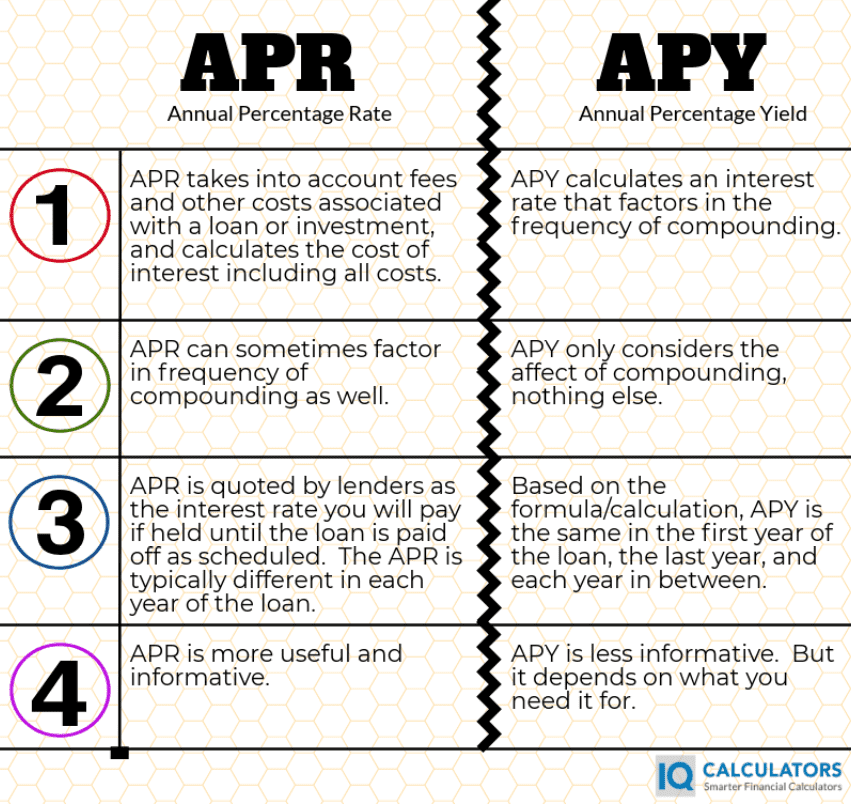# What's The Difference Between APR and APY

APR stands for 'annual percentage rate' and APY stands for 'annual percentage yield'.  So what is the difference between these two terms?

## APR vs APY

The APR is what we will call the effective interest rate that a borrower will pay on a loan, or an investor might receive from an investment, but its less common to use APR when speaking about an investment. The APR is different from the stated interest rate on a loan in that it takes into account a plethora of other factors(primarily fees) in its calculation.  For example, a loan interest rate might be 5 percent, but after the upfront fees and fees that are rolled into the loan amount are accounted for, the APR or effective interest rate may be closer to 5.5 or 6 percent.  In another example, an investor makes an investment that is supposed to deliver a rate of return of 5 percent per year.  But the investor has to pay fees to his broker during the life of the investment.  After fees, his rate or return may only be 4.5 or 4.75 percent.  This would be the investor's APR or effective rate of return.

The APY is the calculated interest rate after accounting for the rate at which the loan or investment compounds interest.  For example, a loan that compounds interest monthly, will have a higher APY than a loan that compounds interest annually.  All else being equal, compounding interest at a higher frequency will always result in a higher annual percentage yield than compounding interest at a lower frequency.

### What Is Compounding Anyway?

For those that don't know, compounding describes the process by which interest is earned and added to the account balance, from which the next interest calculation will be made.  The higher the frequency that interest is calculated and added to the principal balance, the higher the rate of compounding and thus, the higher the annual percentage yield(APY).

## APY Formula

APR and APY formulas are actually very different calculations even though they are very similar concepts in nature.  The APY calculation is stated below.

APY = (1 + r/n)n + 1

Where r = stated interest rate

Where n = frequency of compounding per period

"Period" in the above formula could be per year, per month or whatever is relevant to your situation.

## APR Formula

The APR formula is a little bit different than the APY formula.  APR is much more complicated to calculate as it could potentially need to account for any number of different factors that add expense or cost to the loan.

In order to calculate APR, a person needs to use the IRR(Internal Rate of Return) calculation..  In order to do that, one must account for all cash flows during the life of the loan or investment.  This includes loan payments, loan fees and expenses, and the initial loan amount being lent to the borrower.  As you can tell, this can be overwhelming to do by hand.  The internal rate of return formula should only be done with a financial calculator, excel worksheet...or an online internal rate of return calculator.  Or you can simply use an APR calculator to really help simplify things.

Keep in mind, the APR changes depending on the length of the loan, and APR will differ at any point during the life of the loan.  Upfront fees typically cause the APR to be quite a bit higher in the early years than the laters years in the loan.  However, the APR is generally presented as if the loan were held until it was paid off in the final year as scheduled.

### APR Factors To Potentially Consider

The APR is most typically used to describe an interest rate on a loan and so we will describe the factors that affect APR from that perspective.

First, and most commonly, the APR factors include any loan fees that are either added to the loan, or paid upfront.  This could include loan application fees which are commonly paid upfront, or private mortgage insurance(PMI) that might be added to a mortgage or home loan...just a to name a couple.

Second, APR doesn't commonly factor in the compounding frequency that is used in the APY calculation, but it certainly can in order to give the borrower or lender a more accurate percentage.

Third, the payment frequency is a factor that should be considered as well.  By payment frequency, we mean will the loan payments be made monthly, annually, or quarterly, etc.  The payment frequency of the loan will ultimately affect the interest rate albeit ever so slightly.  Just be aware that the payment frequency can affect the APR of the loan.

Not until a person understands what makes up the APR calculation, can they understand how to lower their APR or how to use APR.## Conclusion

If you haven't figured it out yet, the APR calculation is a much more useful and informative calculation than the APY calculation.  That is why lenders are required to disclose an APR number to borrowers before they sign on the dotted line(via the Truth In Lending).  Keep in mind that the APR has its shortcomings though...primarily that the APR is a number calculated as if the borrower will hold the loan for the entire loan term, most commonly 30 years when speaking about mortgages.  This is true even though most borrowers only own their home for 6 to 7 years on average before moving to their next home.  If fees are paid upfront and/or rolled into the loan, the APR will be much higher in year 6 or 7 than the APR quoted at signing.